# TDoA principles

This page describe the basic principles and functionality of the TDoA modes in the Loco Positioning System.

TDoA means Time Difference of Arrival and is based on the idea of measuring the difference in distance to two anchors as opposed to Two Way Ranging that measures the distance from the Tag to the Anchor. Since time in this context is related to propagation of radio waves, time can be converted to distance using the speed of light.

For instance, if the Tag is located somewhere 2 meters away from Anchor 0 and 3 meters from Anchor 1, the TDoA value (converted to distance) would be 1 meter. Note that in the TDoA modes we do not have access to the distances (3 and 2 meters in this example), only the difference.

Estimating the position from TDoA data is not as straight forward as for Two Way Ranging data and it might seem strange to use this set up, but the main reason is that it is possible to calculate the TDoA data in the Tag by passively listening to the messages sent by the anchors. Since the Tag is only listening, there is no limitation in the system for the number of Tags that can be used concurrently, compare to GPS receivers (Tags) and GPS satellites (Anchors).

## The UWB module

The Ultra Wide Band module that is used in the Anchors as well as the Tags is basically doing two things:

1. It is a radio that can send and receive radio packets that contains a few bytes of data. The packets are very short in time and it is possible to determine when they are received with a very high degree of accuracy.
2. It contains a high resolution timer that is used to get the timestamp of when a packet is sent or received.

## Anchors

The anchors transmit packets as well as listen to packets from other anchors. When an anchor receives a packet from another anchor it includes some of the incomming information in the next outgoing packet. Based on timestamps it also performs some calculations to determine the Time Of Flight (basically the distance to the other anchor) which is also included in the outgoing packets together with the receive timestamp.

## Tags

A TDoA Tag is simply an UWB sniffer. A sniffer can use the data in the packets sent from the anchors together with the packet receive timestamps to calculate its position.

One way of looking at the system is that the Anchors are doing Two Way Ranging with each other and that the possibility to calculate TDoA values for the Tags is merely a nice "side effect".

## Ids

Each anchor has a unique ID that is included in the packets.

## Sequence numbering

Each Anchor maintains a sequence number counter that is included in the sent packets. The counter is increased for each packet that is sent. The sequence number is used by the receiver to detect packet loss.

## Clocks

Each Anchor and Tag has a clock that is driving the high resolution timer. The clock frequency is drifting over time and this error must be compensated for in each unit by continuously tracking the drift of the clocks in the other units. This process is called clock correction.

It is important to understand that each Anchor and Tag has its own clock that is completely un-synchronized to the other clocks. For each clock pulse the local timer counter is increased and the current value of this counter is used for transmit and receive timestamps. Note that the transmit timestamp in a packet is set by the transmitting Anchor, using the clock/counter of the transmitting Anchor. The receive time stamp is set by the receiver (in a different device) using its clock/counter. The two clocks are running at (slightly) different frequencies and the counters will have different values.

One unit does not know the exact state of a remote clock, it can only see it indirectly through the transmit timestamps of the received packets. Further more it does not know exactly how far away the transmitting unit is and thus does not know how long it takes for the packet to travel to the receiver.

The counters in the timers are wrapping which must be handled by the receiver.

## Position data

The absolute position of each anchor is stored in non volatile memory in the Anchor and is included in the transmitted packets.

## Calculating TDoA

This section will explain the theory of how to calculate the TDoA, in English and with drawings. For more details please see the source code that implements one of the modes.

If we consider the 3 last packets sent from anchor 0 and 1: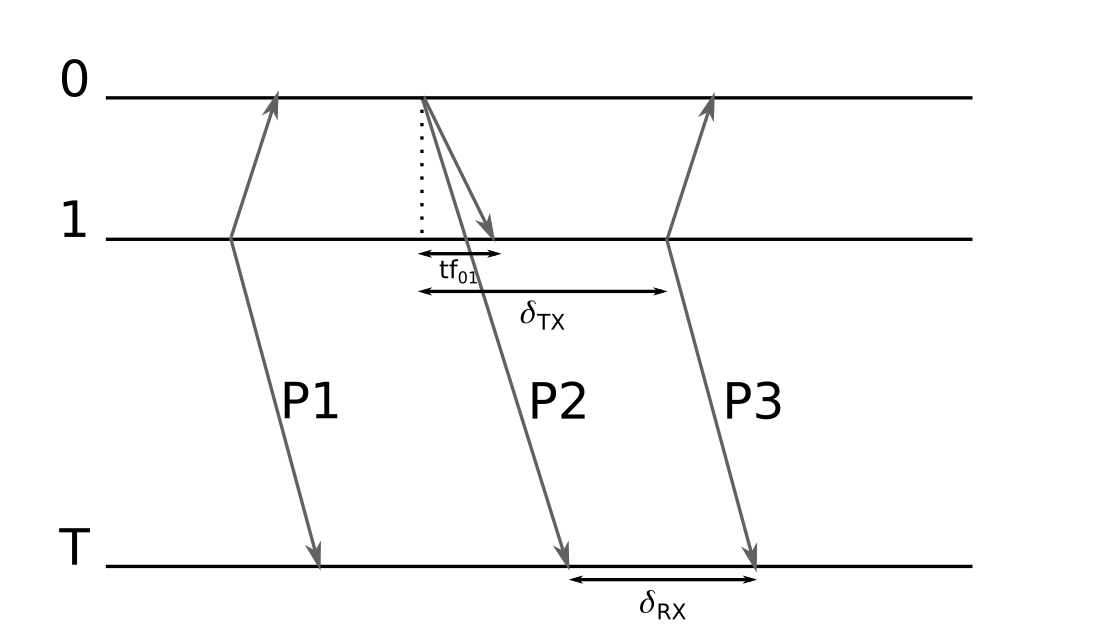We can calculate the difference of time of flight (which is the difference of time of arrival, TDoA) by calculating the difference between δtx and δrx. From the tag's side this can be done by receiving the last packet from each anchor: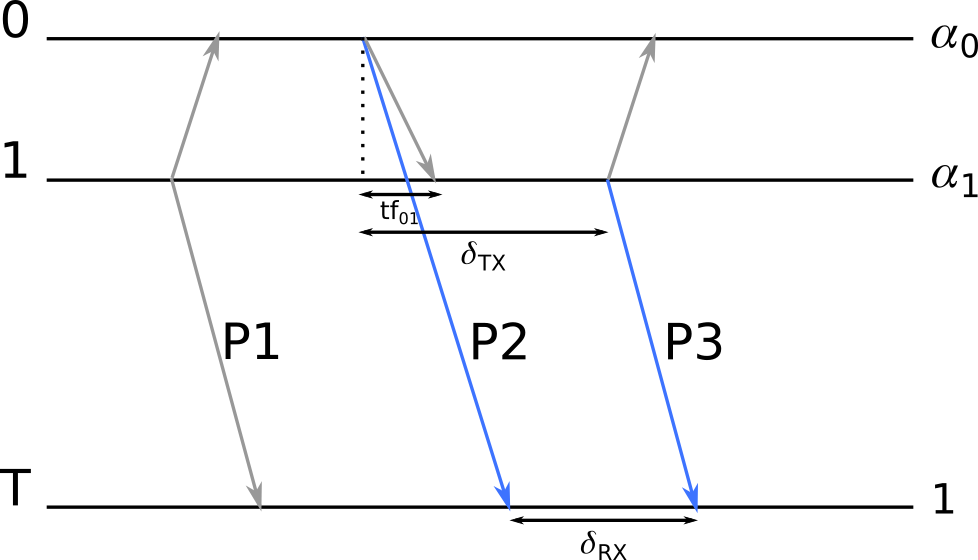δrx is measured when receiving the two packets. δtx can be calculated in the anchor 1 clock if we know the time it takes to fly from 0 to 1: Anchor 1 measures the time of arrival of the packet P2 and so knowing the time it took the packet to arrive to 1, we can calculate when it was sent by 0.

The time of flight between 0 and 1 is measured by the anchors and transmitted in the packets. It is measured using two way ranging: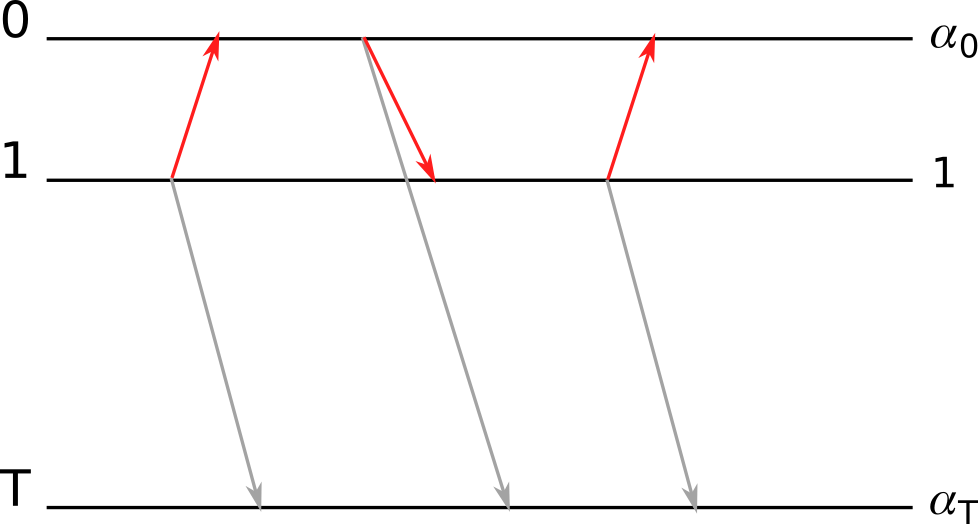Once we know the transmit time of P2 in the anchor 1, we can calculate δtx and δrx. Though δtx is expressed in the Anchor 1 clock. Each node have an imperfect clock and we need to compensate for drift between the clocks. To do so the tag uses two consecutive packet from Anchor 1 to keep track of the clock drift coefficient between its own clock and Anchor 1 clock: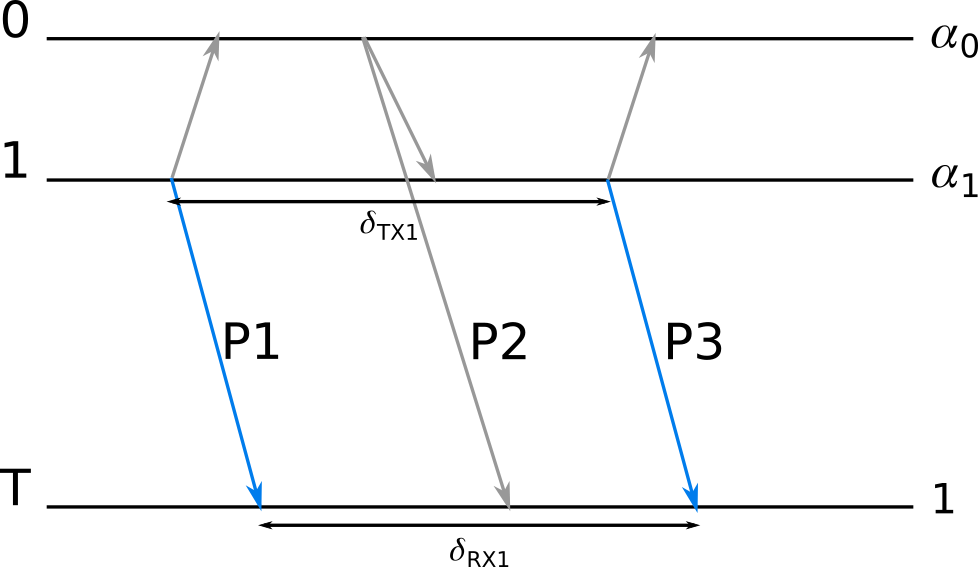Using these two packets we can calculate α₁ = δrx1 / δtx1. We can now calculate TDoA = δrx - (α₁ * δtx).

## Geometry

The geometrical properties of TDoA limits the space where the position can be estimated with a reasonable accuracy.

Consider an example set up where we have three anchors, A0, A1 and A2. This example is in 2D to make it easier to visualize, but the same principles are valid for 3D.

In the first image the Crazyflie (Tag) is located at (0.0, -0.5). The lines where the TDoA is constant are drawn in the figure and the position is where the lines cross. For instance, the TDoA between anchors A0 and A1 is 0.44 (a bit closer to A0 than A1), this means that the Tag could be anywhere on the blue line. The yellow line shows possible positions based on the TDoA value between A0 and A2, while the green line is for TDoA between A1 and A2.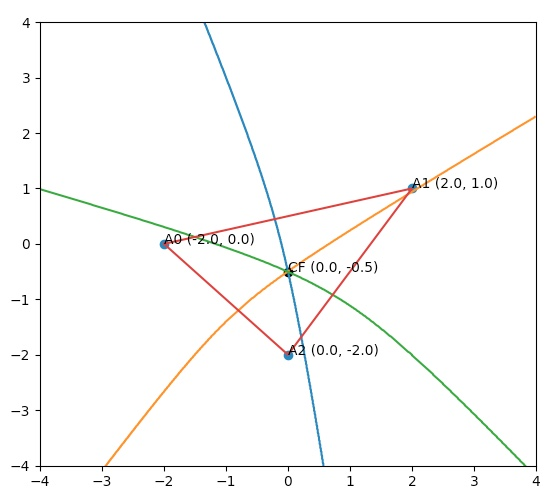In the second image the Tag is further away, at (3.5, -0.3). Note how the lines showing the possible positions are close to parallel in the intersection point, making it harder to estimate a correct possition.The red lines shows the convex hull of the system, basically the space defined by the anchors. A good rule of thumb is that the accuracy of the estimated position is good withing the convex hull but deteriorates quickly when moving away outside.

The measured TDoA values in a real system contains errors and all the TDoA curves will never cross in one single point. The estimated position used in a Tag is instead a point that is "as close as possible" to all the curves, a point where the total error is minimized.